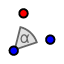# Rotating Around Points: Ex. 17

Topic:
Rotation

## DIRECTIONS:

In the GeoGebra applet below: 1) Create a slider that is an angle. Set Min = 0 degrees. Set Max = 360 degrees. Set Increment = 1 degree. 2) Choose the ROTATE AROUND POINTtool. Highlight a box around point A, point B, and Daffy Duck's pic. Select point C as the point about which to rotate the items you've just selected. For angle, go to the menu on the right and choose . 3) Select the MOVE tool. 4) Move the slider right and left. Note the images of points A and B (denoted as A' and B'). Feel free to move points A and B around as well. After doing all this, please answer the questions that appear below the applet.

## 1.

Let C = (0,0) be the point about which points A and B (and Daffy Duck) are rotated. Place point A at (2, 3) and point B at (5, 1). Set . What are the coordinates (x, y) of the image of A? What are the coordinates (x, y) of the image of B?

## 2.

Let C = (0,0) be the point about which points A and B (and Daffy Duck) are rotated. Place point A at (2, 3) and point B at (5, 1). Set What are the coordinates (x, y) of the image of A? What are the coordinates (x, y) of the image of B?

## 3.

Let C = (0,0) be the point about which points A and B (and Daffy Duck) are rotated. Place point A at (2, 3) and point B at (5, 1). Set . What are the coordinates (x, y) of the image of A? What are the coordinates (x, y) of the image of B?

## 4.

Let (0,0) be the point about which points A and B (and Daffy Duck) are rotated. Suppose the coordinates of point A are now labeled as (x, y). Now even though we don't know what the coordinates of point A are, can you write expressions (in terms of x and/or y) for the coordinates of the image of A under a a) 90 degree counterclockwise rotation about (0,0)? b) 180 degree counterclockwise rotation about (0,0)? c) 270 degree counterclockwise rotation about (0,0)?

When you're done (or if you're unsure of something), feel free to check by watching the quick silent screencast below the applet.